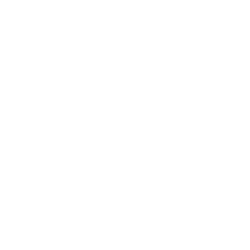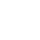## Manipulate an Image with Scripting`var R = 0; var G = 0; var B = 0; if (RLevel > GLevel && RLevel > BLevel) { R = 255; } if (GLevel > BLevel && GLevel > RLevel) { G = 255; } if (BLevel > RLevel && BLevel > GLevel) { B = 255; }`

Here we are first defining the variables R,G and B and setting them to 0 then we have three 'if' statements so for the first one it is basically saying if RLevel is bigger than GLevel and BLevel then set the R value to 255. The same goes for the G value and B value, if in the off chance any of the values are equal then all values will remain 0.

### Step 10:

Now we will create a new color then fill the layer in with this color, using exactly the same commands that we used in step 4 and 5. The code for doing this is:

`var color = new SolidColor(); color.rgb.red = R; color.rgb.green = G; color.rgb.blue = B; docRef_1.selection.selectAll(); docRef_1.selection.fill(color); docRef_1.selection.deselect();`

The only difference is that we called the variable color, just because this could either be red, green or blue; in my case it was blue.

Each command here explains itself fairly well, this process would be done in Photoshop by hitting Ctrl+I then changing the blending mode and opacity manually in the layers panel then hitting Ctrl+E.

### Step 12:

Now we want to add the border, the way I did this was to have this script enlarge the canvas then create a new layer and do a select all and fill this layer white. Before we do this we need to have the script calculate the size of the border depending on the size of the image. After a bit of trial and error I found a good border thickness to be 7.5% of the smaller dimension; either the height or the width. To implement this we first need to find which is smaller the width or the height then to make the border size equal to 7.5% of this. Lastly we have to double this thickness then add it to the width and the height to get the new canvas size. To simplify this slightly I just took 15% rather than 7.5% then I didn't need to double it before I added it on. The code for this stage is:

`var width = docRef_1.width; var height = docRef_1.height; if (width <= height) { var borderSize = Math.round(width * 0.15); } else { var borderSize = Math.round(height * 0.15); } docRef_1.resizeCanvas(width + borderSize, height + borderSize);`

Okay, the first two lines are easy; we are setting a variable for the width which is equal to the document width and a variable for the height which is equal to the document height.

Then we have an 'if' statement which says if the width is smaller or equal to the height then execute the command in the curly brackets. The first part of this command looks familiar; just creating a variable, the second part uses a Math function called round, what this does is to round whatever is in the brackets to the closest integer or whole number. We need this because some of the time 15% of the width will be a decimal number.

Pages: 1 2 3 4 5 6 7 8 9

### One comment on “Manipulate an Image with Scripting”

1.Drake says:

confused